Function Repository Resource:

# FoldRotate

Nest a list of functions around a seed, folding in a list of elements

Contributed by: Daniel Robinson
 ResourceFunction["FoldRotate"][{f1, f2, f3, …}, x, {e1, e2, e3, …}] gives f1[x, e1], then nests the remaining f2, f3, …, folding in the e2, e3, …. ResourceFunction["FoldRotate"][{f1, f2, f3, …}, {e1, e2, e3, …}] is equivalent to ResourceFunction["FoldRotate"][{f1, f2, f3, …}, e1, {e2, e3, …}].

## Details

FoldRotate is a generalisation of Fold, going from a single function in the first argument to a list of functions.
ResourceFunction["FoldRotate"][{f1, f2, , fn}, x, {e1, e2, , en}] yields fn[…f2[f1[x, e1], e2], … en].
FoldRotate[{f}, x, {e1, e2, e3, }] is equivalent to Fold[f, x, {e1, e2, e3, }].
FoldRotate[{f, f, f, …}, x, {e1, e2, e3, }] is equivalent to Fold[{f}, x, {e1, e2, e3, }], albeit slower.
In the case ResourceFunction["FoldRotate"][{f1, , fn}, x, {e1, , em}], if n<m, the functions fi will "rotate" from fn back around to f1. If n>m, only the first m functions will be used in the output.
Like Fold, ResourceFunction["FoldRotate"] can be exited with Throw ».
Unlike Fold, ResourceFunction["FoldRotate"] does not have any operator forms.

## Examples

### Basic Examples (7)

Apply three functions (f, g and h) and three elements (a, b and c) to a seed (x):

 In:=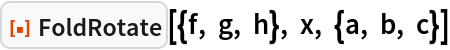Out=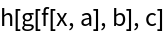Use the 2-argument form to get the same result:

 In:=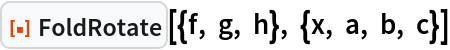Out=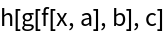If the number of functions is less than the number of elements, the functions will "rotate" round to fill out:

 In:=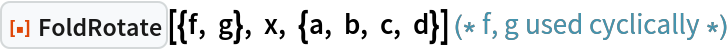Out=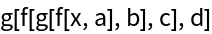If the number of functions is greater than the number of elements, some may not be used:

 In:=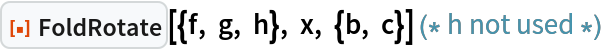Out=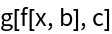Using an empty list of functions will return the list of elements along with the seed:

 In:=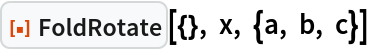Out=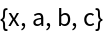Using an empty list of elements and no seed will return an empty list:

 In:=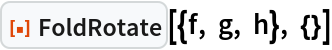Out=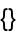Adding a seed effectively prevents the second list from being registered as empty:

 In:=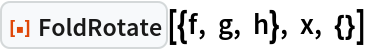Out=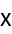### Scope (5)

FoldRotate can take an arbitrary number of functions or elements:

 In:=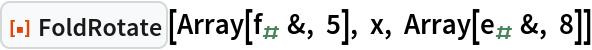Out=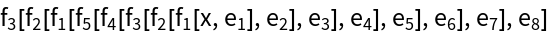FoldRotate can take pure functions. The first slot (#1) refers to the current value (starting with the seed); the second slot (#2) refers to the element being folded in:

 In:=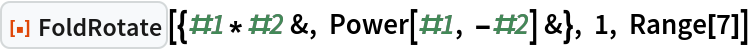Out=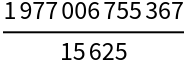Use Sow and Reap to get intermediary results (similar to how FoldList gets intermediary results for Fold):

 In:=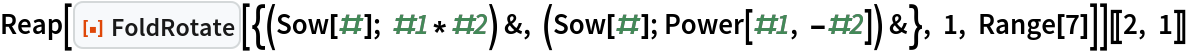Out=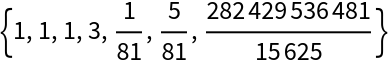An iterator and piecewise function can be used in place of multiple functions:

 In:=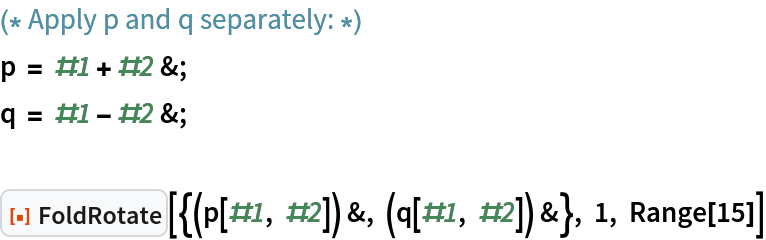Out=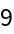In:=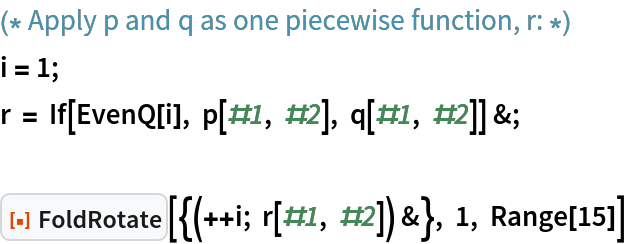Out=FoldRotate can be exited early with Throw. Use FoldRotate (along with Sow and Reap) to generate the nth partial sum in the series 1 + 2 × 3 + 4 × 5 + …:

 In:=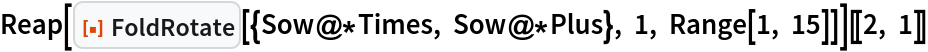Out=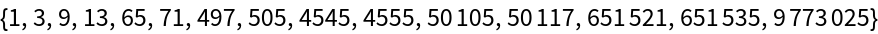Exit early when the partial sum is a multiple of 7:

 In:=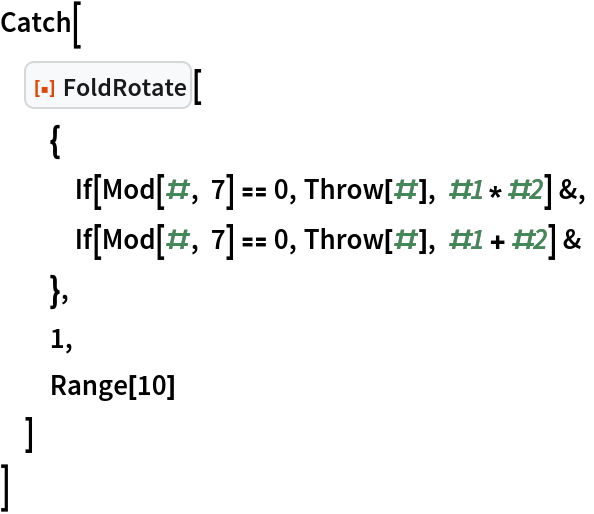Out=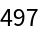### Applications (1)

Calculate alternating totals of a list:

 In:=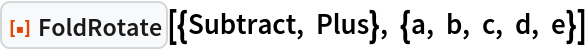Out=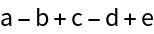In:=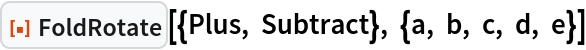Out=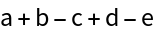### Properties and Relations (3)

FoldRotate can be used to mimic the behaviour of Fold:

 In:=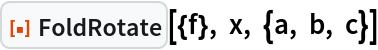Out=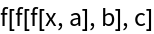In:=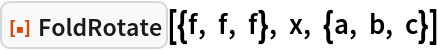Out=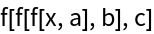In:=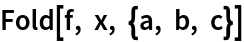Out=In fact, if only one function is provided, FoldRotate (in its 3-argument form) reduces to Fold:

 In:=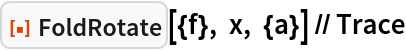Out=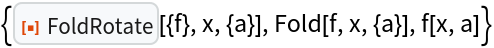In order to return an unevaluated expression, Defer (or HoldForm) must be used at every level:

 In:=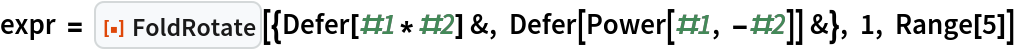Out=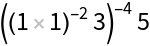Evaluate the expression with a replacement rule:

 In:=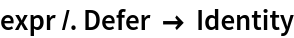Out=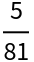FoldRotate is generally slower than Fold, especially when using large number of functions:

 In:=Out=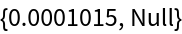In:=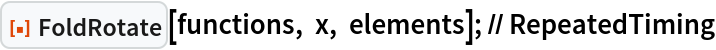Out=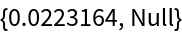### Possible Issues (3)

FoldRotate takes either two or three arguments:

 In:=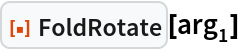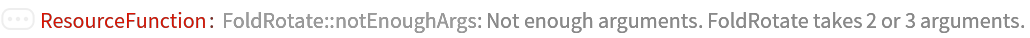Out=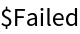In:=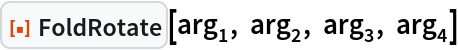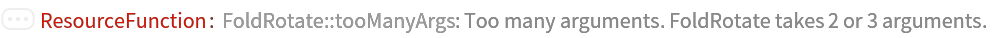Out=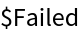The first and last arguments must be lists:

 In:=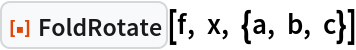Out=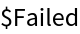In:=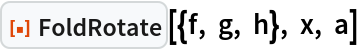Out=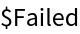Given no arguments at all, FoldRotate will not evaluate:

 In:=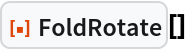Out=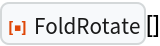### Neat Examples (1)

Create a nesting of frames cycling through three colours:

 In:=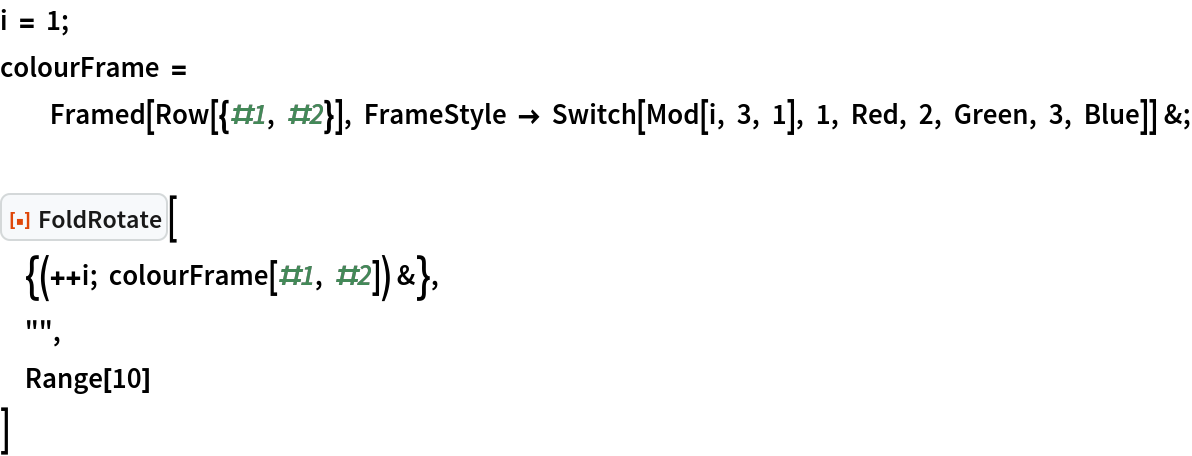Out=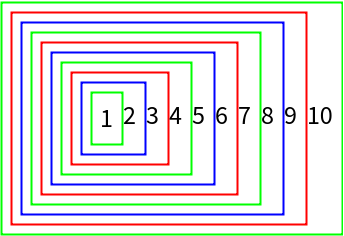Daniel Robinson

## Version History

• 1.0.0 – 16 June 2023